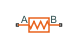# Thermal Resistance

Constant resistance in thermal systems

•Libraries:
Simscape / Foundation Library / Thermal / Thermal Elements

## Description

Thermal resistance is an abstract quantity that relates the thermal conductivity, the heat transfer coefficient, and the radiation coefficient. The Thermal Resistance block lets you model heat transfer in generalized terms, independent of whether it is by conduction, convection, radiation, or a combination thereof.

The heat transfer equation for the Thermal Resistance block is:

`$R\cdot Q=\Delta T$`

where:

• R is the thermal resistance.

• Q is the heat flow rate.

• ΔT is the temperature difference between layers.

Thermal resistance is related to the other heat transfer quantities as follows:

`$R=\frac{D}{k\cdot A}=\frac{1}{h\cdot A}=\frac{1}{r\cdot A\left({T}_{A}^{2}+{T}_{B}^{2}\right)\left({T}_{A}+{T}_{B}\right)}$`

where:

• D is material thickness, that is, distance between layers.

• A is area normal to the heat flow direction.

• k is thermal conductivity of the material.

• h is convection heat transfer coefficient.

• TA and TB are temperatures at ports A and B, respectively.

### Variables

To set the priority and initial target values for the block variables prior to simulation, use the Initial Targets section in the block dialog box or Property Inspector. For more information, see Set Priority and Initial Target for Block Variables.

Nominal values provide a way to specify the expected magnitude of a variable in a model. Using system scaling based on nominal values increases the simulation robustness. Nominal values can come from different sources, one of which is the Nominal Values section in the block dialog box or Property Inspector. For more information, see Modify Nominal Values for a Block Variable.

## Ports

### Conserving

expand all

Thermal conserving port associated with layer A.

Thermal conserving port associated with layer B.

## Parameters

expand all

Thermal resistance value.

## Version History

Introduced in R2017b